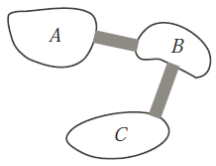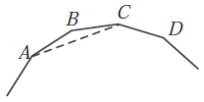# 2009COMC加拿大数学公开赛真题免费下载

### 真题与答案下载### 2009 COMC真题免费下载

Part A共8题，每题5分

Part B共4题，每题10分

#### 部分真题预览：

Part A :

3)Soroosh has 10 coins, each of which is either a quarter (worth 25 cents) or a dime (worth 10 cents). The total value of the dimes is greater than the total value of the quarters. What is the smallest possible number of dimes that he could have?

5)In the diagram, there are three islands labelled A, B and C. Islands A and B are joined by a bridge, as are islands B and C. Maya begins her journey on island A and travels between the islands by bridge only. She records the sequence of islands that she visits. She does not necessarily visit all three islands. If Maya makes 20 bridge crossings in total, how many possible sequences of islands A, B and C could she travel along?6)A polygon is called regular if all of its sides are equal in length and all of its interior angles are equal in size. In the diagram, a portion of a regular polygon is shown. If ∠ACD = 120°, how many sides does the polygon have?Part B :

2)Triangle PQR has vertices P(7,13), Q(19,1) and R(1,1). Point M(4, 7) is the midpoint of PR; the midpoint of PQ is N.

1. Determine the equation of the median of the triangle that passes through points Q and M.
2. Determine the coordinates of G, the point of intersection of RN and QM.
3. Point F is on PR so that QF is perpendicular to PR. Point T is on PQ so that RT is perpendicular to PQ. Determine the coordinates of H, the point of intersection between altitudes QF and RT.
4. Determine which of G and H is closer to the origin.

### 2009 COMC加拿大数学奥赛完整版答案免费下载